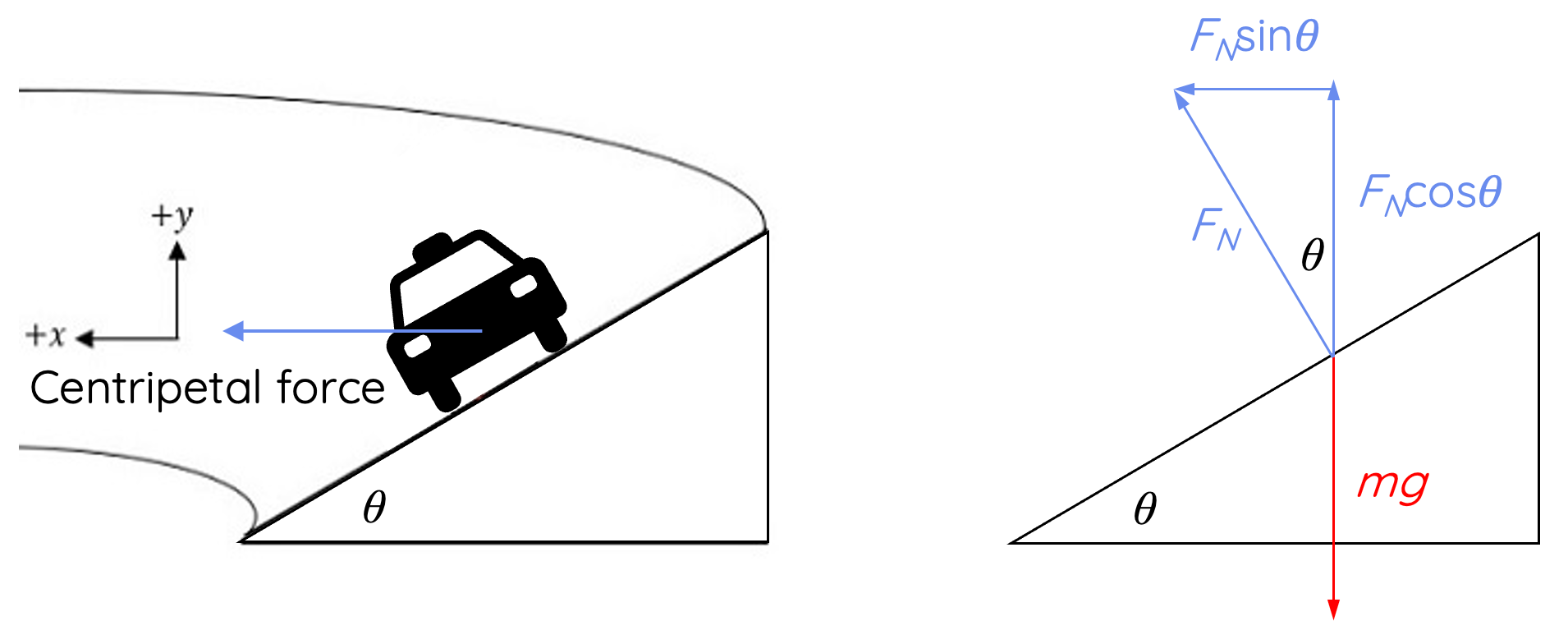# Circular Motion – Objects on Banked Surfaces

This topic is part of the HSC Physics course under the section Circular Motion.

### HSC Physics Syllabus

• analyse the forces acting on an object executing uniform circular motion in a variety of situations, for example:

- cars moving around horizontal circular bends
- a mass on a string
- objects on banked tracks

### Objects Moving in Circular Motion on Banked Surfaces

This video discusses the forces in play in circular motion on banked surfaces without friction. Banked surface scenarios involving friction are discussed separately here.

### Objects on banked surfaces

This scenario is similar to cars driving around a circular bend as identical forces are involved (friction, weight and normal force). However, in this case normal force is not equivalent to weight force so the object is more susceptible to inward force (motion). In addition to velocity, radius and mass, there are more factors which influence the object’s circular motion including the angle of the banked surface to the horizon.

### Forces on a Banked Surface

The first step to approaching circular motion on banked surfaces is to draw a free body diagram:Since the surface is banked at an angle, the direction of normal force is at an angle. This means we must resolve the normal force vector into its horizontal and vertical components.

$$N_x = N\sin{\theta}$$

$$N_y = N\cos{\theta}$$

In contrast to circular motion on a horizontal surface, the horizontal component of normal force also contributes to the centripetal force (net force towards the centre of circular motion).

### Calculating the Ideal Velocity

The ideal velocity required to undergo uniform circular motion on a banked surface can be determined by considering the forces in horizontal and vertical axes.

Forces in horizontal axis:

Equating the horizontal component of normal force (N) to the centripetal force:

(1): Nsintheta=(mv^2)/r

Forces in vertical axis:

Equating the vertical component of the normal force (N) to the weight force (mg):

(2): Ncostheta=mg

Now, by dividing equation (1) by (2), we obtain:

(Nsintheta)/(Ncostheta)=((mv^2)/r)/(mg)

This simplifies into:

tantheta=v^2/(rg)

rg*tantheta=v^2

Finally, we obtain the expression for tangential velocity required to complete uniform circular motion:

v=sqrt(rg*tantheta)

From this expression, the velocity is dependent on

• radius of the circular motion
• acceleration due to gravity
• angle of the banked surface above the horizontal; greater the angle, greater the maximum velocity without the object skidding.

The velocity required to complete circular motion on a banked surface is also lower than a horizontal bend of the same radius.

### Example

A curve of radius 70 m is banked at a 15° angle. At what speed can a car take this curve without assistance from friction?

Solution:

Using the steps of derivation shown above (you must show these steps in an assessment setting):

$$v = \sqrt{rg\tan{\theta}}$$

$$v = \sqrt{(70)(9.8)\tan{15}}$$

$$v = 13.6 \, m \, s^{-1}$$